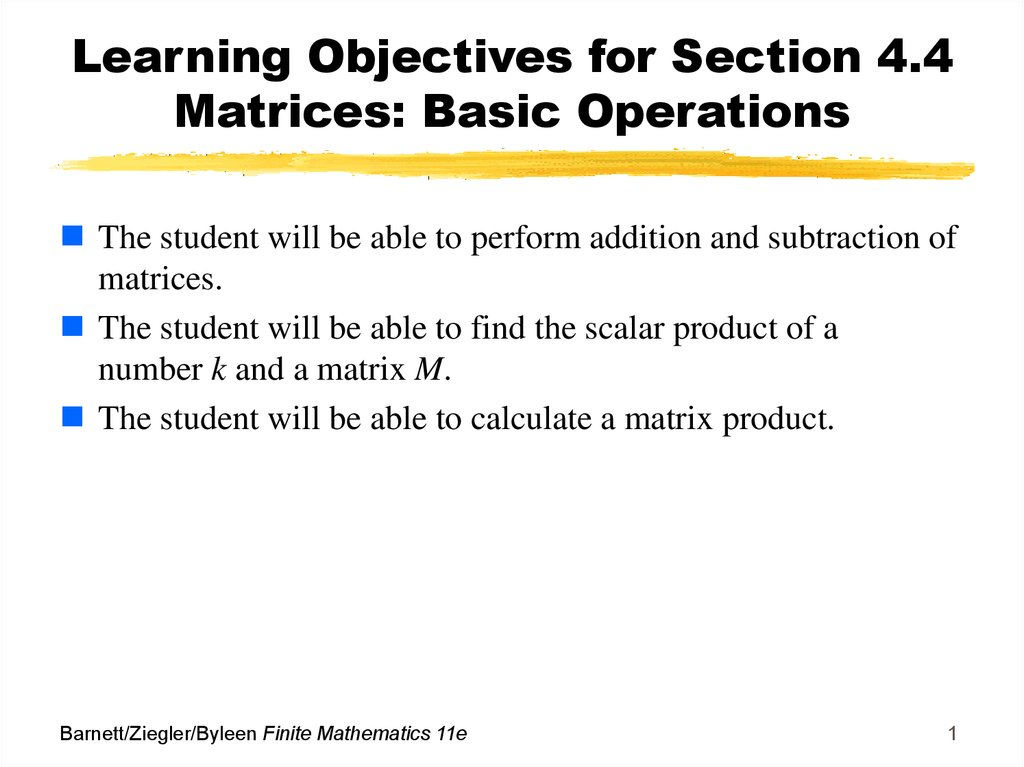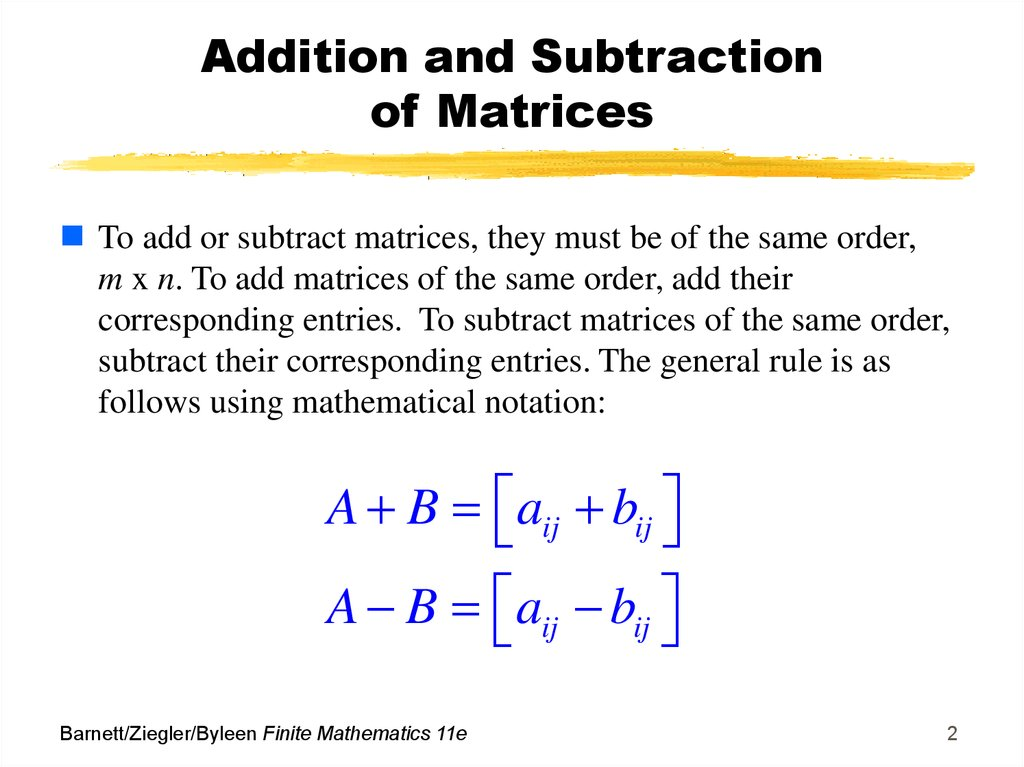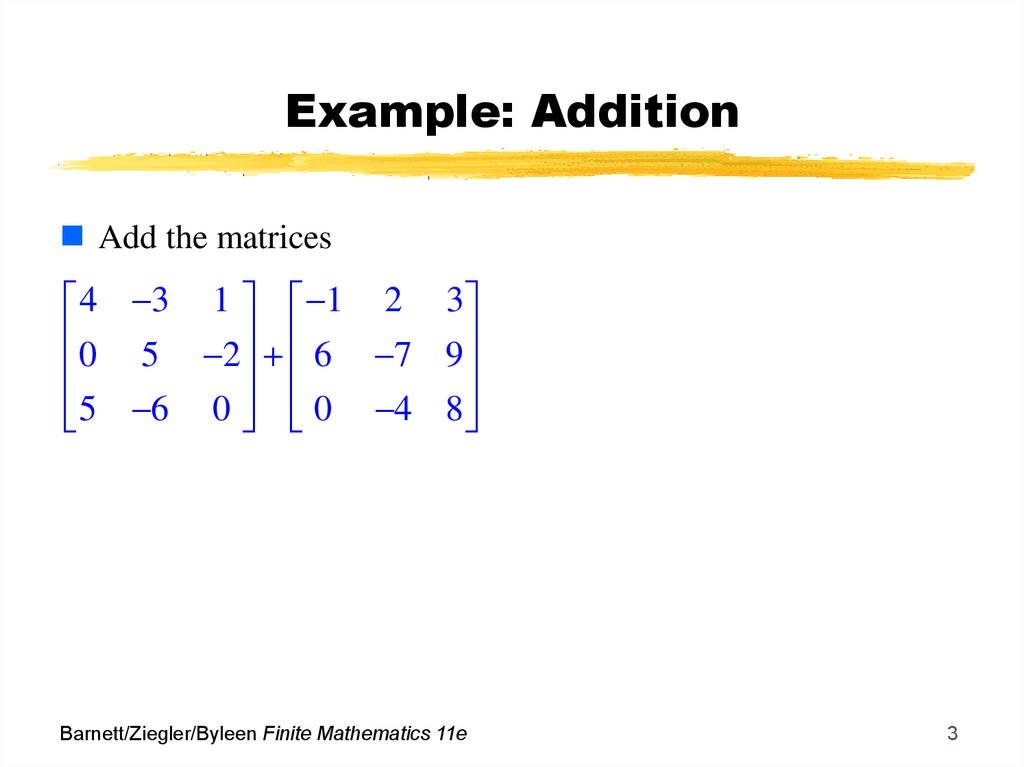# Matrices: Basic Operations

## 1. Learning Objectives for Section 4.4 Matrices: Basic Operations

The student will be able to perform addition and subtraction of
matrices.
The student will be able to find the scalar product of a
number k and a matrix M.
The student will be able to calculate a matrix product.
Barnett/Ziegler/Byleen Finite Mathematics 11e
1

## 2. Addition and Subtraction of Matrices

To add or subtract matrices, they must be of the same order,
m x n. To add matrices of the same order, add their
corresponding entries. To subtract matrices of the same order,
subtract their corresponding entries. The general rule is as
follows using mathematical notation:
A B aij bij
A B aij bij
Barnett/Ziegler/Byleen Finite Mathematics 11e
2

4 3 1 1 2 3
0 5 2 6 7 9
5 6 0 0 4 8
Barnett/Ziegler/Byleen Finite Mathematics 11e
3

4 3 1 1 2 3
0 5 2 6 7 9
5 6 0 0 4 8
Solution: First note that
each matrix has dimensions
of 3x3, so we are able to
result is shown at right:
Barnett/Ziegler/Byleen Finite Mathematics 11e
entries, we have
3 1 4
6 2 7
5 10 8
4

## 5. Example: Subtraction

Now, we will subtract the
same two matrices
4 3 1 1 2 3
0 5 2 6 7 9
5 6 0 0 4 8
=
Barnett/Ziegler/Byleen Finite Mathematics 11e
5

## 6. Example: Subtraction Solution

Now, we will subtract the
same two matrices
4 3 1 1 2 3
0 5 2 6 7 9
5 6 0 0 4 8
Subtract corresponding
entries as follows:
3 2
1 3
4 ( 1)
0 6
5
(
7)
2
9
5 0
6 ( 4) 0 8
=
Barnett/Ziegler/Byleen Finite Mathematics 11e
5 5 2
6 12 11
5 2 8
6

## 7. Scalar Multiplication

The scalar product of a number k and a matrix A is the
matrix denoted by kA, obtained by multiplying each entry of
A by the number k. The number k is called a scalar. In
mathematical notation,
kA kaij
Barnett/Ziegler/Byleen Finite Mathematics 11e
7

## 8. Example: Scalar Multiplication

Find (-1)A, where A =
1 2 3
6 7 9
0 4 8
Barnett/Ziegler/Byleen Finite Mathematics 11e
8

## 9. Example: Scalar Multiplication Solution

Find (-1)A, where A =
1 2 3
6 7 9
0 4 8
Solution:
1 2 3
(-1)A= -1 6 7 9
0 4 8
1 2 3 1 2 3
( 1) 6 7 9 6 7 9
0 4 8 0 4 8
Barnett/Ziegler/Byleen Finite Mathematics 11e
9

## 10. Alternate Definition of Subtraction of Matrices

The definition of
subtraction of two real
numbers a and b is
a – b = a + (-1)b or
“a plus the opposite of b”.
We can define subtraction
of matrices similarly:
Barnett/Ziegler/Byleen Finite Mathematics 11e
If A and B are two matrices
of the same dimensions,
then
A – B = A + (-1)B,
where (-1) is a scalar.
10

## 11. Example

The example on the right
illustrates this procedure for
two 2x2 matrices.
Barnett/Ziegler/Byleen Finite Mathematics 11e
1 2 2 1
3 4 3 1
1 2
2 1
( 1)
3 4
3 1
1 2 2 1
3
4
3
1
1 3
0 5
11

## 12. Matrix Equations

Example: Find a, b, c, and d so that
a b 2 1 4 3
c d 5 6 2 4
Barnett/Ziegler/Byleen Finite Mathematics 11e
12

## 13. Matrix Equations

Example: Find a, b, c, and d so that
a b 2 1 4 3
c d 5 6 2 4
Solution: Subtract the matrices on the left side:
a 2 b 1 4 3
c 5 d 6 2 4
Use the definition of equality to change this matrix equation
into 4 real number equations:
a-2=4
a=6
b+1=3
b=2
Barnett/Ziegler/Byleen Finite Mathematics 11e
c + 5 = -2
c = -7
d-6=4
d = 10
13

## 14. Matrix Products

The method of
multiplication of matrices
is not as intuitive and may
seem strange, although
this method is extremely
useful in many
mathematical applications.
Matrix multiplication was
introduced by an English
mathematician named
Arthur Cayley (1821-1895).
We will see shortly how
matrix multiplication can be
used to solve systems of
linear equations.
Barnett/Ziegler/Byleen Finite Mathematics 11e
14

## 15. Arthur Cayley 1821-1895

Introduced matrix multiplication
Barnett/Ziegler/Byleen Finite Mathematics 11e
15

## 16. Product of a Row Matrix and a Column Matrix

In order to understand the general procedure of matrix
multiplication, we will introduce the concept of the product of
a row matrix by a column matrix.
A row matrix consists of a single row of numbers, while a
column matrix consists of a single column of numbers. If the
number of columns of a row matrix equals the number of rows
of a column matrix, the product of a row matrix and column
matrix is defined. Otherwise, the product is not defined.
Barnett/Ziegler/Byleen Finite Mathematics 11e
16

## 17. Row by Column Multiplication

Example: A row matrix consists of 1 row of 4 numbers so this
matrix has four columns. It has dimensions 1 x 4. This matrix
can be multiplied by a column matrix consisting of 4 numbers
in a single column (this matrix has dimensions 4 x 1).
1x4 row matrix multiplied by a 4x1 column matrix. Notice the
manner in which corresponding entries of each matrix are
multiplied:
Barnett/Ziegler/Byleen Finite Mathematics 11e
17

## 18. Example: Revenue of a Car Dealer

A car dealer sells four model types: A, B, C, D. In a given
week, this dealer sold 10 cars of model A, 5 of model B, 8 of
model C and 3 of model D. The selling prices of each
automobile are respectively \$12,500, \$11,800, \$15,900 and
\$25,300. Represent the data using matrices and use matrix
multiplication to find the total revenue.
Barnett/Ziegler/Byleen Finite Mathematics 11e
18

## 19. Solution using Matrix Multiplication

We represent the number of each model sold using a row
matrix (4x1), and we use a 1x4 column matrix to represent the
sales price of each model. When a 4x1 matrix is multiplied by a
1x4 matrix, the result is a 1x1 matrix containing a single
number.
12,500
11,800
10(12,500) 5(11,800) 8(15,900) 3(25,300) 387,100
10 5 8 3
15,900
25,300
Barnett/Ziegler/Byleen Finite Mathematics 11e
19

## 20. Matrix Product

If A is an m x p matrix and B is a p x n matrix, the matrix
product of A and B, denoted by AB, is an m x n matrix whose
element in the i th row and j th column is the real number
obtained from the product of the i th row of A and the j th
column of B. If the number of columns of A does not equal
the number of rows of B, the matrix product AB is not defined.
Barnett/Ziegler/Byleen Finite Mathematics 11e
20

## 21. Multiplying a 2x4 matrix by a 4x3 matrix to obtain a 2x3

The following is an illustration of the product of a 2x4 matrix
with a 4x3. First, the number of columns of the matrix on the
left must equal the number of rows of the matrix on the right,
so matrix multiplication is defined. A row-by column
multiplication is performed three times to obtain the first row
of the product: 70 80 90.
Barnett/Ziegler/Byleen Finite Mathematics 11e
21

## 22. Final Result

Barnett/Ziegler/Byleen Finite Mathematics 11e
22

## 23. Undefined Matrix Multiplication

Why is the matrix multiplication below not defined?
Barnett/Ziegler/Byleen Finite Mathematics 11e
23

## 24. Undefined Matrix Multiplication Solution

Why is the matrix multiplication below not defined?
The answer is that the left matrix has three columns but the
matrix on the right has only two rows. To multiply the second
row [4 5 6] by the third column, 3 , there is no number to pair
with 6 to multiply.
7
Barnett/Ziegler/Byleen Finite Mathematics 11e
24

## 25. Example

1
B= 3
2
3 1 1
Given A =
2
0
3
6
5
4
Find AB if it is defined:
3 1 1
2 0 3
1
3
2
Barnett/Ziegler/Byleen Finite Mathematics 11e
6
5
4
25

## 26. Solution

Since A is a 2 x 3 matrix
and B is a 3 x 2 matrix, AB
will be a 2 x 2 matrix.
1. Multiply first row of A by
first column of B:
3(1) + 1(3) +(-1)(-2)=8
2. First row of A times
second column of B:
3(6)+1(-5)+ (-1)(4)= 9
3. Proceeding as above the
final result is
Barnett/Ziegler/Byleen Finite Mathematics 11e
1
3 1 1
2 0 3 3
2
=
6
5
4
8 9
4 24
26

## 27. Is Matrix Multiplication Commutative?

Now we will attempt to multiply the matrices in reverse
order: BA = ?
We are multiplying a 3 x 2 matrix by a 2 x 3 matrix. This
matrix multiplication is defined, but the result will be a 3 x 3
matrix. Since AB does not equal BA, matrix multiplication is
not commutative.
1
3
2
6
3 1 1
=
5
2 0 3
4
Barnett/Ziegler/Byleen Finite Mathematics 11e
15 1 17
1 3 18
2 2 14
27

## 28. Practical Application

Suppose you a business owner and sell clothing. The
following represents the number of items sold and the cost for
each item. Use matrix operations to determine the total
revenue over the two days:
Monday: 3 T-shirts at \$10 each, 4 hats at \$15 each, and
1 pair of shorts at \$20.
Tuesday: 4 T-shirts at \$10 each, 2 hats at \$15 each, and
3 pairs of shorts at \$20.
Barnett/Ziegler/Byleen Finite Mathematics 11e
28

## 29. Solution of Practical Application

Represent the information using two matrices: The product of
the two matrices gives the total revenue:
Unit price of
each item:
3 4
10 15 20 4 2
1 3
Qty sold
of each
item on
Monday
Qty sold of
each item on
Tuesday
Then your total revenue for the two days is = [110 130]
Price times Quantity = Revenue
Barnett/Ziegler/Byleen Finite Mathematics 11e
29